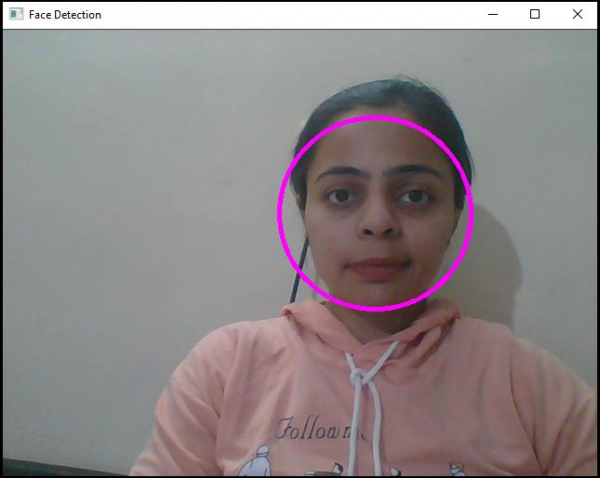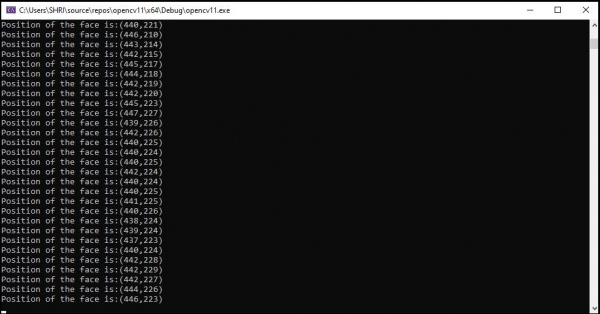# How to track the face in real-time in OpenCV using C++?

OpenCVC++Server Side ProgrammingProgramming

We will learn how to track the face in real-time in OpenCV. This program is same to the previous program and difference is that we used ellipse instead of rectangle to identify the face and we also used additional 'cout' statement to show the co-ordinate of the face in the console window.

The following program to detect human face in real-time −

## Example

#include<iostream>
#include<opencv2/highgui/highgui.hpp>
#include<opencv2/imgproc/imgproc.hpp>
//This header includes definition of 'rectangle()' function//
#include<opencv2/objdetect/objdetect.hpp>
#include<string>
using namespace std;
using namespace cv;
int main(int argc, char** argv) {
Mat video_stream;//Declaring a matrix hold frames from video stream//
VideoCapture real_time(0);//capturing video from default webcam
namedWindow("Face Detection");//Declaring an window to show the result//
string trained_classifier_location = "C:/opencv/sources/data/haarcascades/haarcascade_frontalface_alt.xml";//Defining the location our XML Trained Classifier in a string//
vector<Rect>faces;//Declaring a rectangular vector named faces//
while (true) {
faceDetector.detectMultiScale(video_stream, faces, 1.1, 4, CASCADE_SCALE_IMAGE, Size(30, 30));//Detecting the faces in 'image_with_humanfaces' matrix//
for (int i = 0; i < faces.size(); i++){ //for locating the face
Point center(faces[i].x + faces[i].width * 0.5, faces[i].y + faces[i].height * 0.5);//getting the center of the face//
ellipse(video_stream, center,Size(faces[i].width * 0.5, faces[i].height * 0.5), 0, 0, 360, Scalar(255, 0, 255), 4, 8, 0);//draw an ellipse on the face//
int horizontal = (faces[i].x + faces[i].width * 0.5);//Getting the horizontal value of coordinate//
int vertical=(faces[i].y + faces[i].width * 0.5);//Getting the vertical value of coordinate//
cout << "Position of the face is:" << "(" << horizontal << "," << vertical << ")" << endl;
//Showing position in the console window//
}
imshow("Face Detection", video_stream);
//Showing the detected face//
if (waitKey(10) == 27){ //wait time for each frame is 10 milliseconds//
break;
}
}
return 0;
}

## Output